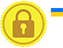# if statement in javascript

if statement is used to check the specified condition and if it is correct if block statements is executed and if false execute the next statement.

Try Yourself

### Execution flow of above example - image explanation

• Variable a and b is created and value 50 and 30 are assigned (var a=50; var b=30;).
• A condition (a>b) is checked, and it is true (50>30).
• Alert statement inside if block is executed (alert("'a' is bigger than 'b'");).
• if statement ends and the control execute the next statement (alert("Finished");)

# if..else statement in javascript

if statement is used to check the specified condition and if it is correct if block statements is executed and if false execute the else block statements.

Try Yourself

### Execution flow of above example - image explanation

• Variable a and b is created and value 50 and 300 are assigned (var a=50; var b=300;).
• A condition (a>b) is checked, and it is false (50>300), so the control moves from if block to else block.
• Alert statement inside else block is executed (alert("'a' is lesser than 'b'");).
• if else statement ends and the control execute the next statement (alert("Finished");)

# if..else if...else statement in javascript

In a program after if statement any number of else if statement can be followed.

In here if statement is used to check the specified condition and if it is correct if block statements is executed, and if false it checks the else if condition if it is correct it execute the else if block statements, if there is an else if statement again the process goes on and if no conditions are satisfied the else block will be executed.

Try Yourself

### Execution flow of above example - image explanation

• Variable a and b is created and value 50 and 300 are assigned (var a=50; var b=300;).
• A condition (a>b) is checked, and it is false (50>300), so the control moves from if block to else if block.
• A condition (a
• Alert statement inside else if block is executed (alert("'b' is bigger than 'a'");).
• else if statement ends and the control execute the next statement (alert("Finished");)